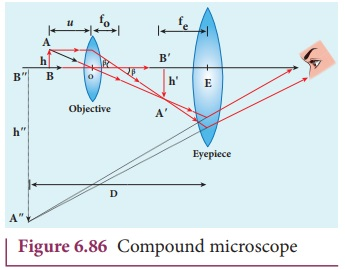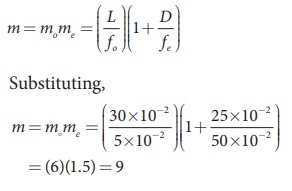Home | | Physics 12th Std | Compound microscope

# Compound microscope

Magnification of compound microscope

Compound microscope

The diagram of a compound microscope is shown in Figure 6.86. The lens near the object, called the objective, forms a real, inverted, magnified image of the object. This serves as the object for the second lens which is the eyepiece. Eyepiece serves as a simple microscope that produces finally an enlarged and virtual image. The first inverted image formed by the objective is to be adjusted close to, but within the focal plane of the eyepiece so that the final image is formed nearly at infinity or at the near point. The final image is inverted with respect to the original object. We can obtain the magnification for a compound microscope.

## Magnification of compound microscope

From the ray diagram, the linear magnification due to the objective is,From the Figure 6.85, tan ╬▓ = h/f0 = hŌĆ▓/L , thenHere, the distance L is between the first focal point of the eyepiece to the second focal point of the objective. This is called the tube length L of the microscope as fo and fe are comparatively smaller than L.

If the final image is formed at P (near point focussing), the magnification me of the eyepiece is,The total magnification m in near point focusing is,If the final image is formed at infinity (normal focusing), the magnification me of the eyepiece is,The total magnification m in normal focusing is,### EXAMPLE 6.42

A microscope has an objective and eyepiece of focal lengths 5 cm and 50 cm respectively with tube length 30 cm. Find the magnification of the microscope in the (i) near point and (ii) normal focusing.

### Solution

f0 = 5cm = 5├Ś10ŌłÆ2 m; fe = 50cm = 50├Ś10ŌłÆ2 m;

L = 30cm = 30├Ś10ŌłÆ2 m; D = 25cm = 25├Ś10ŌłÆ2 m

(i) The total magnification m in near point focusing is, m = mome(ii) The total magnification m in normal  focusing is, m = momeTags : Optical Instruments , 12th Physics : UNIT 7 : Wave Optics
Study Material, Lecturing Notes, Assignment, Reference, Wiki description explanation, brief detail
12th Physics : UNIT 7 : Wave Optics : Compound microscope | Optical Instruments